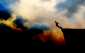# Differential Equation, DE

## Eliminate the Arbitrary Constants

Please do leave a solution so I can study it, thank you!

Obtain the differential equation by eliminating the arbitrary constants.
1. Cxsiny+x^(2)y=Cy
2. y=Ae^(-3x)-Be^(2x)+Cx^3
3. Ay=e^(Bx^2)

## Differential Equation: $y' = x^3 - 2xy$, where $y(1)=1$ and $y' = 2(2x-y)$ that passes through (0,1)

Can anyone solve this D. E.?

y' = x^3 - 2xy, where y(1)=1

and

y' = 2(2x-y) that passes through (0,1)## Help please: $(1+e^x y+x e^x y) dx + (x e^x + 2) dy=0$

(1+e^(x) y+x e^(x) y) dx + (x e^(x) + 2) dy=0

## Arbitrary constant

y=x^2+C1e^2x+C2e^3x

Thank you again

## Families of Curves: family of circles with center on the line y= -x and passing through the origin

Find the diff equation of family of circles with center on the line y= -x and passing through the origin.

THANK YOU :)## DE: $(x²+4) y' + 3 xy = x$

(x²+4) y' + 3 xy = x

## Differential Equations: $(r - 3s - 7) dr = (2r - 4s - 10) ds$

The topic is Additional Topics in Ordinary DE of the first order.

(r-3s-7)dr=(2r-4s-10)ds

## Differential Equations: $(x - 2y - 1) dy = (2x - 4y - 5) dx$

Coefficient Linear in Two Variables? Help please.

(x-2y-1)dy=(2x-4y-5)dx## DIIFERENTIAL EQUATION: $(x^2 + y^2) dx + x (3x^2 - 5y^2) dy = 0$

( x^2 + y^2 ) dx + x (3x^2 - 5y^2 ) dy = 0## Differential EQNS: $y \, dx = \left[ x + (y^2 - x^2)^{1/2} \right] dy$

ydx = x + √ y^2 - x^2 dy

## Pages